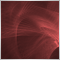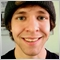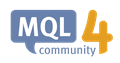# How to do this in MQL4?232

Hi there,
Hope you are doing well.

I want to detect minimum variable among several variables and add 10 to it.
How can I do this in the quickest way?

For example: a=2, b=5, c=9, d=11;
in the above example, I want to find "d" and make it d=21;6642

HosseinKOGO:

Hi there,
Hope you are doing well.

I want to detect minimum variable among several variables and add 10 to it.
How can I do this in the quickest way?

For example: a=2, b=5, c=9, d=11;
in the above example, I want to find "d" and make it d=21;

so you really mean the maximum variable then.....

you need to at least try posting your code attempt, then you may find some here willing to help you get it right.232

Paul Anscombe #:

so you really mean the maximum variable then.....

you need to at least try posting your code attempt, then you may find some here willing to help you get it right.

I have no idea how to do it efficiently and simple.
I have just this idea,Is it just the best idea of doing this?:

```int index=0;
double a;
for(int i=0; i<12; i++)
{
if(MathMax(a[i],a[i+1])==a[i+1]) {index=i+1;}
}
a[index]=a[index]+10;```

But I thought maybe there is a better logic to do this so we have easily access to the second maximum, third maximum etc.271

HosseinKOGO #:

I have no idea how to do it efficiently and simple.
I have just this idea,Is it just the best idea of doing this?:

But I thought maybe there is a better logic to do this so we have easily access to the second maximum, third maximum etc.

Fill your Array with the required values, then use ArraySort().ArraySort - Array Functions - MQL4 Reference
• docs.mql4.com
ArraySort - Array Functions - MQL4 Reference232

Jeremie Courchesne #:

Fill your Array with the required values, then use ArraySort().